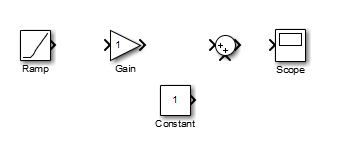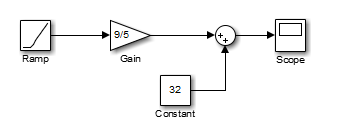Documentation

## Model a Simple Equation

To model the equation that converts Celsius temperature to Fahrenheit

```TF = 9/5(TC) + 32 ```

First, consider the blocks needed to build the model:

• A Ramp block to input the temperature signal, from the Sources library

• A Constant block to define a constant of 32, also from the Sources library

• A Gain block to multiply the input signal by 9/5, from the Math Operations library

• A Sum block to add the two quantities, also from the Math Operations library

• A Scope block to display the output, from the Sinks library

Next, gather the blocks into your model window.Assign parameter values to the Gain and Constant blocks by opening (double-clicking) each block and entering the appropriate value. Then, click the button to apply the value and close the dialog box.

Now, connect the blocks.The Ramp block inputs Celsius temperature. Open that block and change the Initial output parameter to 0. The Gain block multiplies that temperature by the constant 9/5. The Sum block adds the value 32 to the result and outputs the Fahrenheit temperature.

Open the Scope block to view the output. Now, choose Run from the Simulation menu to run the simulation. The simulation runs for 10 seconds.